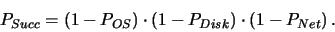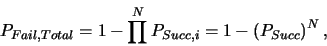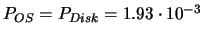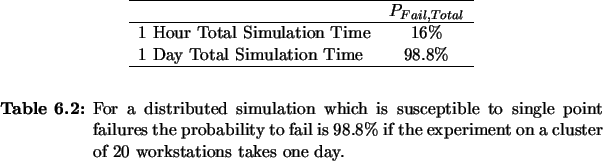Next: 6.2.3 Load Polling Up: 6.2 Distributed Computing Previous: 6.2.1 Workload Distribution

## 6.2.2 Robustness

Distributed computation takes place in a quite complicated technical system. Aside from the stability of the hardware and the operating system itself, distributed computation adds one more source of system failures which is the network connections. Likewise to the complexity of the system, also the probability of system failures rises. Additionally, the longer the overall simulation takes, the more likely is the occurrence of a failure within that period.

In order to get a feeling for the stability that can be achieved let us briefly sketch a case study of distributed computation on a cluster of workstations. Assuming the probability of a hardware or operating system failure is POS, the probability of failure due to disk storage shortage is PDisk, and a network failure occurs with a probability of PNet. Thus the probability for the successful completion of a simulation on a single machine isWhereas the probability to fail for an ensemble of N simulations which are computing in parallel isassuming that all parts of the distributed simulation are exposed to equal risk and an individual failure invalidates the entire simulation. A reasonable assumption for values of POS and PDisk is one failure per month and one failure per week for network failures PNet which is equivalent to, andfailures per hour.

Table 6.2 shows the resulting failure probabilities for two experiments taking one hour's time and one day, respectively, under the assumption that (sub-) processes are computing optimally balanced on a cluster of 20 workstations.Table 6.2 makes clear that parallel and distributed computation on a local area network results in a fairly unstable system unless special measures are taken in order to improve stability. This is particularly true for large scale simulation experiments such as optimizations, which can take up to a week's time or even longer.Next: 6.2.3 Load Polling Up: 6.2 Distributed Computing Previous: 6.2.1 Workload Distribution
Rudi Strasser
1999-05-27